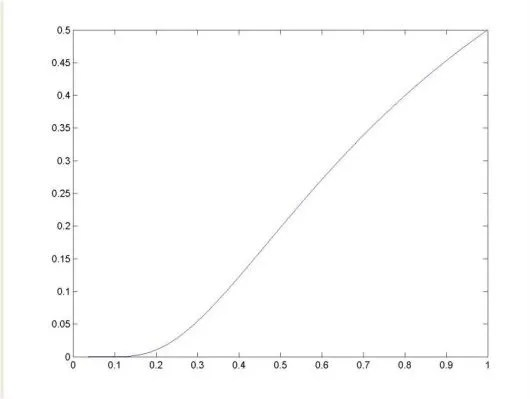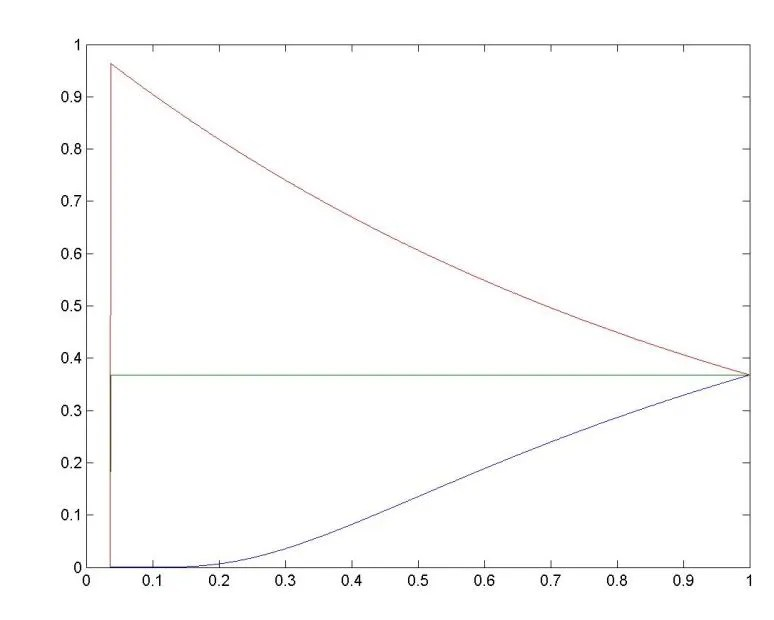# College Math Teaching

## April 26, 2011

### On “0 to the 0’th power”: UPDATED

Filed under: calculus, derivatives, media, popular mathematics — collegemathteaching @ 2:26 am

UPDATED: I’ve extended this discussion to the cases in which the limit functions are pathological and corrected an error.

My friends over at the popular blog Ask a Mathematician, Ask a Physicist did a great post a while ago addressing one of their readers’ questions: What is 0^0?

The reason this question is a head-scratcher is that our rules about how exponents work seem to yield two contradictory answers. On the one hand, we have a rule that zero raised to any power equals zero. But on the other hand, we have a rule that anything raised to the power of zero equals one. So which is it? Does 0^0 = 0 or does 0^0 = 1?

Well, I asked Google and according to their super-official calculator, the answer is unambiguous: […]

Indeed, the Mathematician at AAMAAP confirms, mathematicians in practice act as if 0^0 = 1. But why? Because it’s more convenient, basically. If we let 0^0=0, there are certain important theorems, like the Binomial Theorem, that would need to be rewritten in more complicated and clunky ways. Note that it’s not even the case that letting 0^0=0 would contradict our theorems (if so, we could perhaps view that as a disproof of the statement 0^0=0). It’s just that it would make our theorems less elegant. Says the mathematician:

“There are some further reasons why using 0^0 = 1 is preferable, but they boil down to that choice being more useful than the alternative choices, leading to simpler theorems, or feeling more “natural” to mathematicians. The choice is not “right”, it is merely nice.”

I am curious as to who this “mathematician” is and how well the author of the above article understood what he heard. Here is a fact: if$0^0 \neq 1$, then many basic Theorems of calculus would be wrong.

Of course, is is an elementary calculus exercise to show that$lim_{x \rightarrow 0+} x^x = 1$ and the graph seems to confirm this:(graph is a screenshot of a SCILAB graph)

But one might ask: what if the exponent approaches zero at a greater or lesser rate than the base? For example, what are:$lim_{x \rightarrow 0+} (ln(x+1))^{x}$ or$lim_{x \rightarrow 0+} (x)^{ln(x+1)}$?

UPDATE The following is designed for people who teach calculus; the pace might be too quick for a student who is just learning. At the end of the post I’ll put the details. Back to the post:

Let’s take a look. Suppose$f$ and$g$ are both differentiable analytic at 0 and$f(0)=g(0)=0$.

Then$lim_{x \rightarrow 0+} f(x)^{g(x)} = exp(-((g(x))^2 f'(x))/(f(x)g'(x)))$ by an elementary application of L’Hopital’s rule. So we should examine what is in the exponential exponent:$-((g(x))^2 f'(x))/(f(x)g'(x)) = -g(x) (g(x)/f(x))(f'(x)/g'(x))$.

The product of the ratios$(g(x)/f(x))(f'(x)/g'(x))$ will prove to be the key.

Now use the definition of derivative and the fact that both$f$ and$g$ vanish at zero to simplify this product:$(f'(x)/g'(x)) = lim_{x \rightarrow 0}((f(x)-f(0))/(x-0))/(g(x)-g(0))/(x-0)=lim_{x \rightarrow 0}(f(x)/(g(x))$.
Hence$lim_{x \rightarrow 0+}-((g(x))^2 f'(x))/(f(x)g'(x)) =$$lim_{x \rightarrow 0+} -g(x) (g(x)/f(x))(f(x)/g(x))$$= lim_{x \rightarrow 0+} -g(x) = 0$

Hence$lim_{x \rightarrow 0+} f(x)^{g(x)} = exp(0) = 1$

Note: can you spot the error in my deleted “proof”?

I’ll do it right this time:$-((g(x))^2 f'(x))/(f(x)g'(x)) = -g(x) (g(x)/f(x))(f'(x)/g'(x))$.

The product of the ratios$(g(x)/f(x))(f'(x)/g'(x))$ will prove to be the key. Now exploit the fact that both$f$ and$g$ are analytic at zero and have a Taylor series expansion: say$f(x) = \Sigma^{\infty}_{k=m}a_kx^k$ and$g(x) = \Sigma^{\infty}_{j=n}b_jx^j$
Then$f'(x) = \Sigma^{\infty}_{k=m}ka_kx^{k-1}$ and$g(x) = \Sigma^{\infty}_{j=n}jb_jx^{j-1}$
Now look at the ratio$(g(x)/f(x))(f'(x)/g'(x))$.
This is easier to see if we write the ratio out term by term: the numerator of the fraction is:$(b_n x^n + b_{n+1} x^{n+1} + b_{n+2} x^{n+2}...)(m a_m x^{m-1} + (m+1) a_{m+1} x^{m}...)$
The denominator is:$(n b_n x^{n-1} + (n+1) b_{n+1} x^{n}...)(a_m x^{m} + a_{m+1} x^{m+1}...)$
Note: we can assume that there is no constant term in the Taylor expansion.
Now we can factor out$(x^n x^{m-1} = x^{n+m-1})$ from the numerator and$(x^{n-1} x^m) = x^{n+m-1}$ from the denominator to obtain${(b_n + b_{n+1}x +..)(m a_m + (m+1) a_{m+1}x+..)}/{(n b_n + (n+1) b_{n+1}x +..)( a_m + a_{m+1}x+..)}$ which equals$(m b_n a_m)/(n b_n a_m)$ at$x = 0$ (of course we can assume that$b_n, a_m \neq 0$
Therefore$lim_{x \rightarrow 0+}-g(x) (g(x)f'(x))/(g'(x)f(x)) = 0$ as required and the result follows.

Conclusion: the speed of approach to zero doesn’t really matter, so long as the functions are analytic.

UPDATE: A non-analytic case:

Of course, we might have the case when, say,$f$ approaches zero but fails to be analytic. Then interesting things can happen.
Here is a graph which shows$f(x) = exp(-1/x^2)$ and$g(x) = ln(1+x)$ The above proof doesn’t work as$f(x)$ is not analytic at$x = 0$; indeed$f'(0) = 0$ but the Taylor series is valid at$x = 0$ only. In fact, in this case$g(x)ln(f(x)) = ln(x+1)(-1/x^2)$ which approaches$-\infty$ as$x$ approaches zero. Hence our$f(x)^{g(x)} = 0$ in this case…but we had to use a somewhat pathological function.Note: we can get different limits by playing with the$exp(-1/x^2)$ example. In fact:$\lim_{x\rightarrow 0+}(\exp (-\frac{1}{x^{k}}))^{x^{m}}=$$\lim_{x\rightarrow 0+}\exp (-\frac{x^{m}}{x^{k}})=\left\{ \begin{array}{c} \exp (0)=1\text{ if }m>k \\ \exp (-1)=e^{-1}\text{ if }m=k \\ \exp (-\infty )=0\text{ if }mThis is a Matlab generated example with$exp(-1/x^2)$ with the exponents$x, x^2, x^3$. Note the struggle with round-off error.